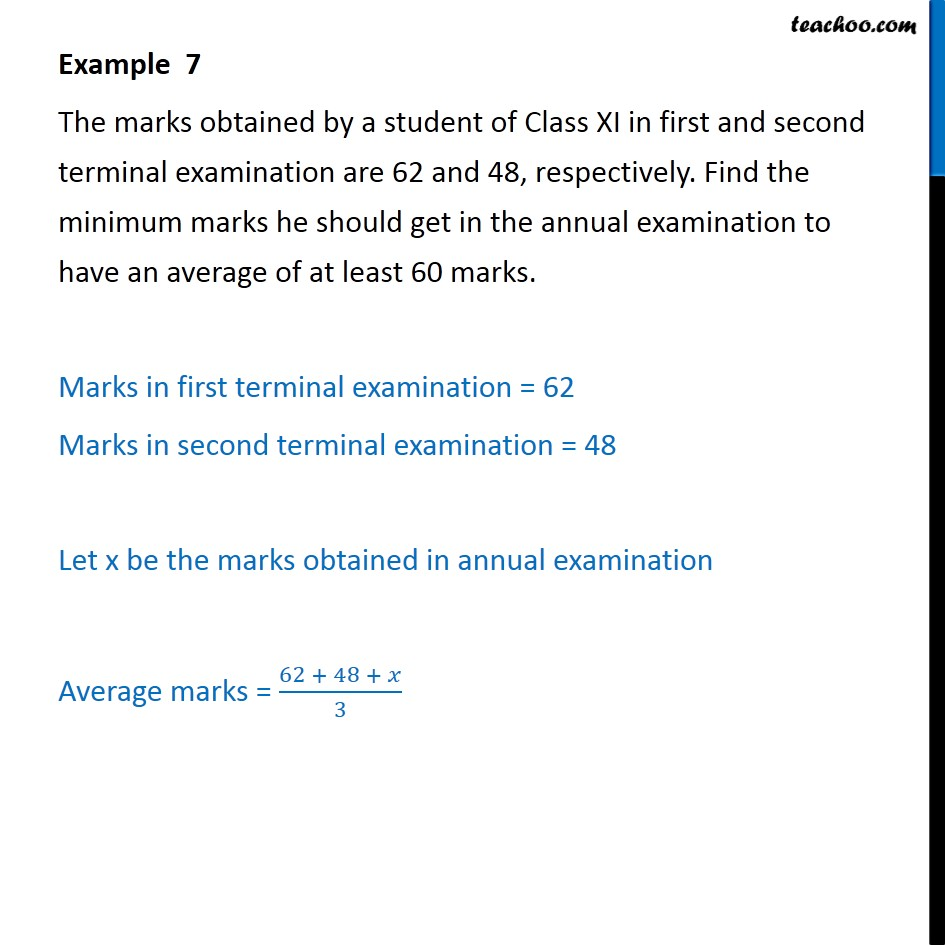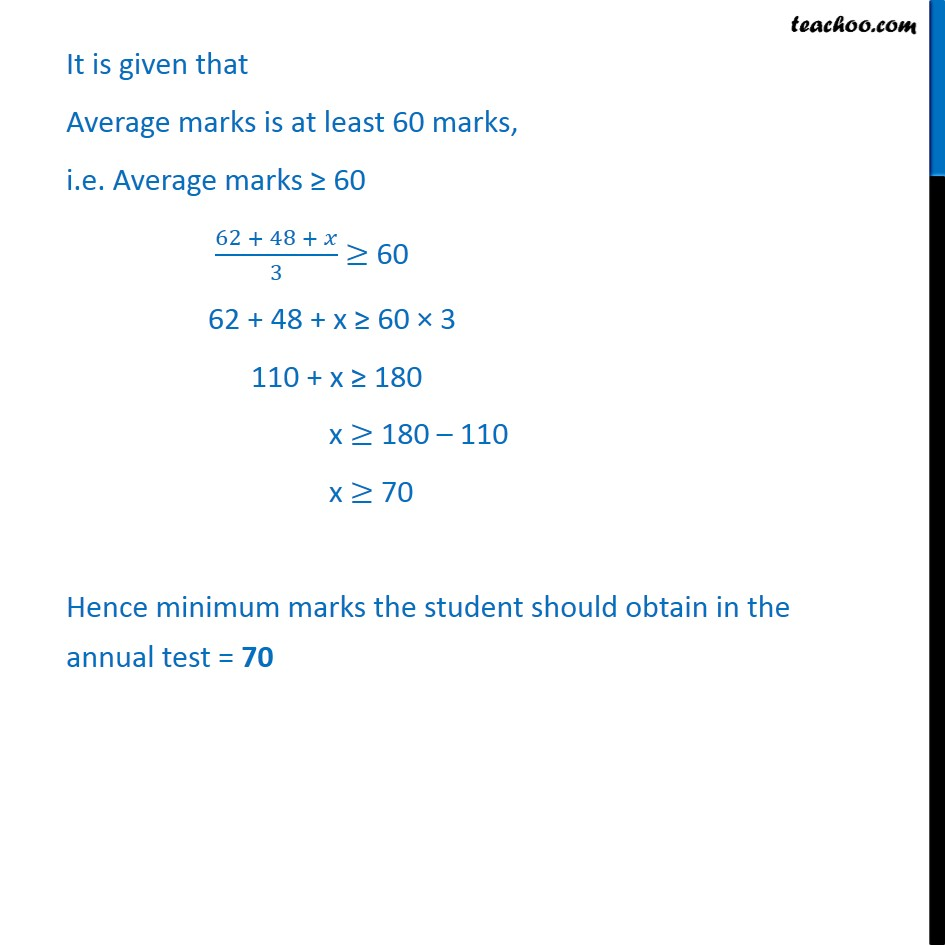Subscribe to our Youtube Channel - https://you.tube/teachoo

1. Chapter 6 Class 11 Linear Inequalities
2. Concept wise
3. Solving inequality - Statement questions

Transcript

Example 7 The marks obtained by a student of Class XI in first and second terminal examination are 62 and 48, respectively. Find the minimum marks he should get in the annual examination to have an average of at least 60 marks. Marks in first terminal examination = 62 Marks in second terminal examination = 48 Let x be the marks obtained in annual examination Average marks = ﷐62 + 48 + 𝑥﷮3﷯ It is given that Average marks is at least 60 marks, i.e. Average marks ≥ 60 ﷐62 + 48 + 𝑥﷮3﷯ ≥ 60 62 + 48 + x ≥ 60 × 3 110 + x ≥ 180 x ≥ 180 – 110 x ≥ 70 Hence minimum marks the student should obtain in the annual test = 70

Solving inequality - Statement questions